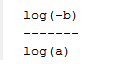Home MathLab Solve Equations With Unknown Coefficients With Matlab

# Solve Equations With Unknown Coefficients With Matlab

We have so far been working with numbers, totally ignoring one of the most rewarding ability Matlab put to our use: the possibility to work with symbolic expressions.

In many college algebraic courses, it is taught how to use and simplify equations with symbolic expressions, where one of the major task is to be learn how to express one symbol with respect to others. Here we will attempt to use Matlab to solve some problems we lately worked on using real numbers.

## Example 1

Let’s consider the following equation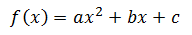We all know that this is second order polynomial equation and we know how to solve it. Let’s try using Matlab to solve this very equation as it is, assuming we don’t know what the value of the coefficients are.

The code

```syms a b c x
f = a*x^2 + b*x + c
solve(f)```

Which returns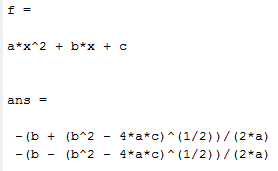The code

`pretty(ans)`

Which returns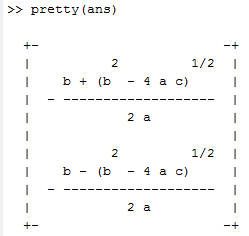Which we all remember from basic algebra.

If you would like to solve the equation with respect to a, you can state it like this

`solve(f,a)`

Which returns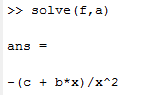## Example 2

Let’s now use the following equation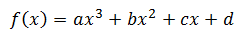Just like the second order polynomial equation in example 1, this will be like it

The code

```syms a b c d x;
f = a*x^3 + b*x^2 + c*x + d;
l = solve(f);
pretty(l)```

Which returns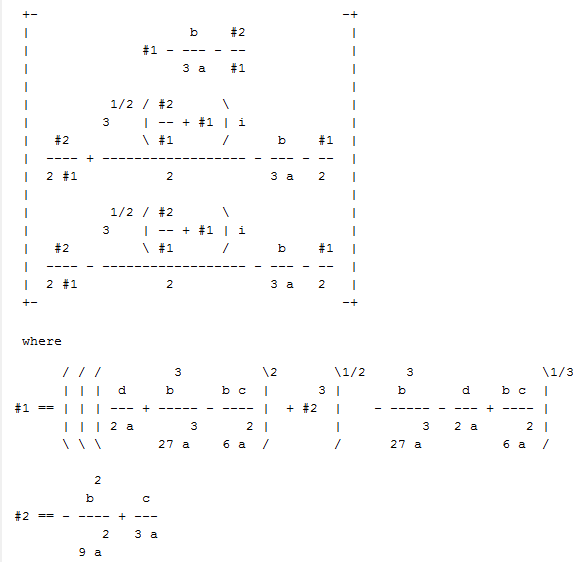## Example 3

This is also a nice method you can make use of, to help you remember a formula. Let’s consider the following Matrix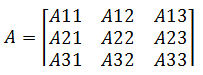Let’s find the determinant of A

The code

```syms A11 A12 A13 A21 A22 A23 A31 A32 A33
A=[A11 A12 A13 ; A21 A22 A23 ; A31 A32 A33]
l = det(A)```

Which returns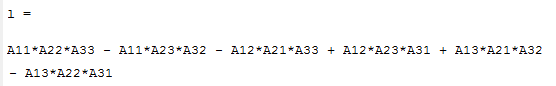## Example 4

Let’s do some Matrix operation with the following matrices: Addition and Multiplication.

##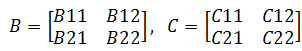the code

```syms B11 B12 B21 B22 C11 C12 C21 C22;
B = [B11 B12 ; B21 B22];
C = [C11 C12 ; C21 C22];
Mul = B*C;
Mul```

Which returns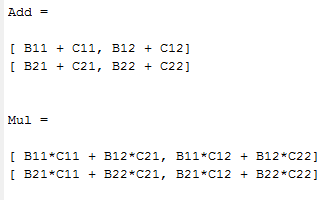## Example 5

Let’s end this session with solving the following equation.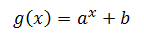The code

```syms a b x
g = a^x + b;
l = solve(g);
pretty(l)```

Which returns